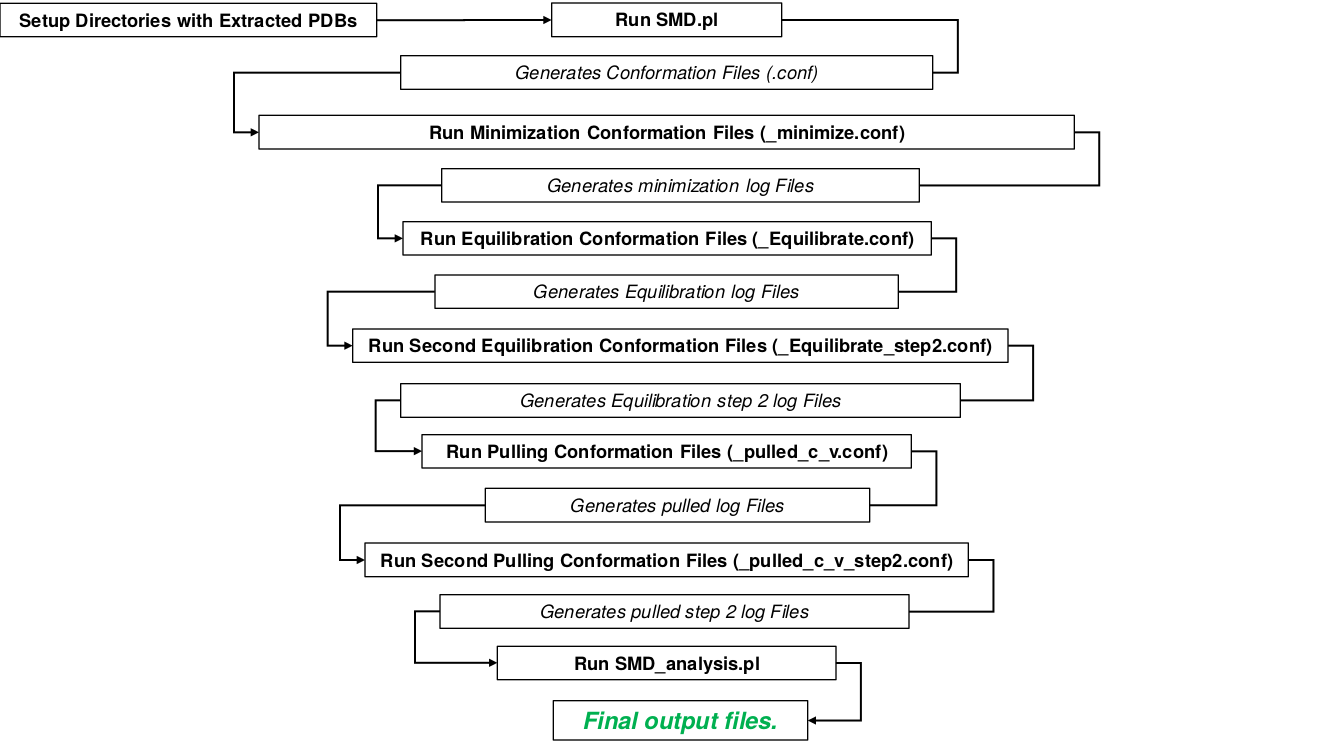Using Steered Molecular Dynamic Tension for Assessing Quality of Computational Protein Structure Models

## Steered Molecular Dynamic Tension as a Method for Determining Computational Protein Structure Model Quality

#### Below are the scripts and data sets used in this work. The tables below are broken up into 3 categorizes, the first table consists of the scripts and data sets used as well as a README file for how to run scripts. The second table is an example input pdb, and the third table contains example output files. When running the perl scripts, it is assumed that pdb files are in a directory named 'pdb'. See README for details.

 File About pdb.tar PDB data set of casp models used in the study, organized by target number. There are a total of 36 target sets with 2976 models in total. Models have names T0###TS###_#.pdb, where # is an integer between 0 and 9. Once untared, run SMD.pl, then run namd simulations. SMD.pl Perl script for setting up NAMD steered molecular dynamics simulations. Runs on all .pdb files in a directory called ./pdb/ SMD.pm Subroutines for SMD.pl SMD_analysis.pl Perl script for the analysis of log files from SMD simulations. Formats force-time curves and finds Peak data. SMD_analysis.pm Subroutines for SMD_analysis.pl README.txt How to set up and run the perl scripts. parameter files par_all36_prot.prm CHARMM36 parameter file for proteins used. toppar_water_ions_namd.str Parameter file for water and ions.
 Example Input Example Pdb Input Example input pdb. Place this file in a directory called ./pdb/T0644/ and run SMD.pl in your ./perl directory.
 Example Output minimize.log An example minimization output log file. This is the output from running namd2 model_minimize.conf as decribed in the README. Equilibrate.log An example equilibration output log file. This is the output from running namd2 model_Equilibrate.conf as decribed in the README. Equilibrate_step2.log An example step 2 equilibration output log file. This is the output from running namd2 model_Equilibrate_step2.conf as decribed in the README. pulled_c_v.log An example pulling output log file. This is the output from running namd2 model_pulled_c_v.conf as decribed in the README. pulled_c_v_step2.log An example step2 pulling output log file. This is the output from running namd2 model_pulled_c_v_step2.conf as decribed in the README. Force.dat An example force curve. Plot data with gnuplot or excel. This kind of file is generated by SMD_analysis.pl. Force_smooth.dat An example smoothed force curve. Plot data with gnuplot or excel. This kind of file is generated by SMD_analysis.pl. This is the data that is used to determine the break force. Force_smooth.dat An example output of the Model GDT_TS and Force from SMD_analysis.pl

## Work Flow